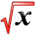# Inverse FunctionsThis article is part of the MathHelp Tutoring Wiki

If f-1 is the inverse of function f then (f-1 o f)=x or f-1(f(x))=x.

Example: Prove g(x)=5/(x-1) is an inverse of f(x)=1+(5/x)

f(g(x))=1+(5/(5/(x-1)))

f(g(x))=1+(x-1)

f(g(x))=1+x-1=x

Example: Find the inverse function of f given by the following,

f(x) = (x-3)2 for x>=3 (this has to hold so that the inverse function does exist)

The function is written in the following format,

y = (x - 3)2

As a result, there are two possible solutions given below:

x - 3 = sqrt(y) or x - 3 = - sqrt(y)

However since sqrt(y) is always larger than zero and x >= 3, the first solution is chosen,

x - 3 = sqrt(y), therefore, x = sqrt(y) + 3;

f-1 (y) = sqrt(y) + 3 or f-1(x) = sqrt(x) + 3;